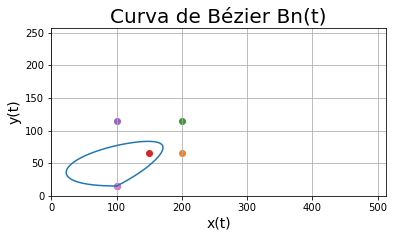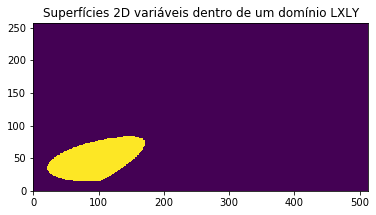# Parametric Equations

Hi.

Rhino is new for me, but I’m used to Python. I read on the internet that it is possible to create scripts inside the software, so here it go my question:

I would like to know if there is any way for me to get all my model’s parametric equations, like NURBS, Splines, etc.

I supose that it would be a great number of equations, but I believe that it wouldnt be a real problem.

it will not be possible to extract the information as you believe. there was a topic discussing your request here a while ago.

1 Like

Hi,

The answer is yes, however your scope is rather broad, can you elaborate on what you would like to extract from the model.
Best would be an example so we have something concrete to relate to.

-Willem

1 Like

how? manually or grasshopper? ok, since this is under scripting i guess everything is allowedi better shut it then.

Sure.

Basically what I want to create is a matrix which contains 0 or 1: if my matrix’s index is ‘out’ of my geometry, 0. If it is ‘inside’, 1.

The reason why I would like to have all the parametric equations that creates my model is simple: with them it would be very easy to tell my matrix’s index where it got to be 0 or 1.

I created some content in Jupyter Notebook (with Python), but only with simple objects, for example: a solid which the shape is like a Bézier curve.

Here’s my Bézier, which I use as limit of my solid:Here’s my matrix, where purple is 0 and yellow is 1:Hi @mombandre ,
Is this just a single case problem or do you need a general solution which works for any curve?
Is your curve based on some mathematical principle or are the curves more or less random?

There is a function to test if a point is inside a closed planar curve:
https://developer.rhino3d.com/api/RhinoScriptSyntax/#curve-PointInPlanarClosedCurve
Maybe that helps

1 Like

If I understand you correctly, you want to check which interval in the XY plane is contained within the your curve. Then you would add contained `x,y` pairs as `1` in a Matrix and `0` as not contained.

I can provide you a way to approximate a given curve mathematically in a iterative fashion.

Let me know if this will help

Hi @Jess.

Definitely what I need is a general solution. Today I only draw simple shapes, but my goal is to be able to recognize any shape, for example a whole ship.

About the second question, my curve need to be known. I wish I could draw anything and my script could read this object and give me all the equations that has build the object.

I know, it’s totally scary and it there is a massive work to go, so I want to focus in an environment that my goal would be reachable.

Exactly.

Yes, I would be very grateful!

It is possible to approximate your curves with Fourier transformations but to determine if any points are inside your curves it takes more… Since you mention a ship, Rhino has nice volume tools, hydro-statics and many more advanced tools for simulation etc. Maybe you can tell us a bit more about the problem you want to solve before we reinvent the wheel… ehm… foils1 Like

@mombandre

Please see this were is explains the Chaikins Algorithm, and which I used to implement it as well. Hehehe first one that appears in google search and also a very straight forward paper.

To use it, copy and paste the following code in a C# component in Grasshopper.

``````
private void RunScript(List<Point3d> pts, int Iterations, ref object A)
{
// More iterations = more precision
// Input a list of points, which would be the contol points
A = ChaikinsAlgorithm(pts, Iterations);
}

public Polyline ChaikinsAlgorithm(List<Point3d> cPoints, int iterations)
{
// Include startPt
Point3d st = cPoints;

// Include EndPt
Point3d end = cPoints[cPoints.Count - 1];

int i = 0;
while (i < iterations)
{
List<Point3d> newCPs = new List<Point3d>();
for (int j = 0; j < cPoints.Count; j++)
{
if(j < cPoints.Count - 1)
{
// Implementing Chaikins method... 3/4 , 1/4 stuff
Point3d quarter = ((cPoints[j + 1] - cPoints[j]) * 0.25) + cPoints[j];
Point3d threeFourths = ((cPoints[j + 1] - cPoints[j]) * 0.75) + cPoints[j];
}

}

newCPs.Insert(0, st);
newCPs.Insert(newCPs.Count - 1, end);
cPoints = newCPs;
i++;
}

return new Polyline(cPoints);
}

``````

I think perhaps the easiest way to go is instead of trying to find out the exact formula of an input curve ( sounds very complicated) you can use an approximation method given a tolerance. This would be much easier and perhaps much more manageable. That will fit in the idea of calculus, right? How small is enough? limits… etc.

2 Likes

Thanks for the code!

For sure, there is another ways which are simplier, but I need the precision.

The idea behind getting parametric equations is getting closer to the solid exact dimensions as I’m resizing my matrix. It works like a mesh.

Imagine: if I draw a circle and try to transform it geometry to a matrix 0 or 1, positioning it in the middle and not changing it size. Bigger matrix means less error: if my matrix is 9x9, it would look like a square, but if I resize it to a 900x900, it get better.

But anyway, thanks for everything!

Yeah, for sure there is many ways to get into a curve. The magic behind the NURBS is that we only need to pick the points and the equation is generated. I just wanted that equation!

I would use Rhino just for draw a solid and then get these equations. After I would keep my path through another code.

Thanks!

Sorry, I may be confused but I do not understand. Which points do you pick? Bézier points?
Also, if you have parametric equations how can you determine if a point is inside the graph?
You have a solid in 3D? What are you trying to solve?

I understand.

Imagine I’m going to draw a foil with a Bézier or a Spline. My first step would be pick some random points (at this point the software already show me a smoothly curved line between the points). After, I would start organizing the points until the curve reach my desired shape (now the software got the equations that describes exactly my foil, otherwise that would not be a curve connecting them!). That’s the equation I want.

First, let’s imagine a matrix behind a cartesian graph. Pretty much the same as a grid. Imagine that in the middle of every square formed by the grid, there is a point.

These points correspond to each index i,j of my matrix.

Index per index (in a loop) I’m going to ask if the point is inside the surface that was created by the Bézier or Spline curve.

Not yet. I’m currently working with surfaces, which are easier. But a parametric surface’s equation is similar to a parametric curve’s equation.

I’m trying to transform a solid into a matrix!

Ok this you can do with the function I’ve already posted:

With that I think you do not need any parametric equations or implicit functions to create a matrix.
Is this for a CFD simulation?

Edit: You’ll find NURBS math on wikipedia, and there are also nice explanations here in this forum.

1 Like

Yep!

Thank you very much for the help! I’m going to work a little bit, sure more questions are going to surge.

Cheers!